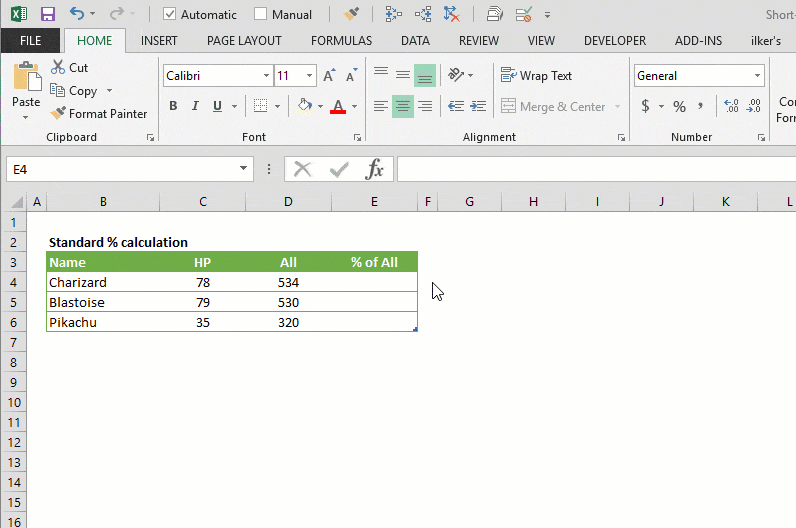A percentage is a number or ratio that represents a fraction of 100, and this representation is used widely from interest rates to risk analysis, or really in any application when the contribution of a specific item to the whole is to be highlighted. In this article, we’re going to show you how to calculate percentage in Excel and also go over some tips and examples.# Standard Percentage Calculation

Since we use a decimal (base 10) system, multiplying the ratio of two numbers by a hundred is enough to find the percentage representation.

Percentage = ( numerator / denominator ) * 100

For example, 3 of 4 equals to 75 / 100 which is 75% percentage-wise.

# How to Calculate Percentage in Excel

Percentage calculations are even easier, as you do not need to multiply the fraction by 100. To calculate percentage in Excel, by simply applying a number format and Excel will display the number as a percentage value.

Percentage = ( numerator / denominator )

Upon entering your formula, select the cell and click on the % icon from the HOME tab to apply a percentage number format. Alternatively, you can apply a percentage format from the Format Cells dialog just like applying any other format type.